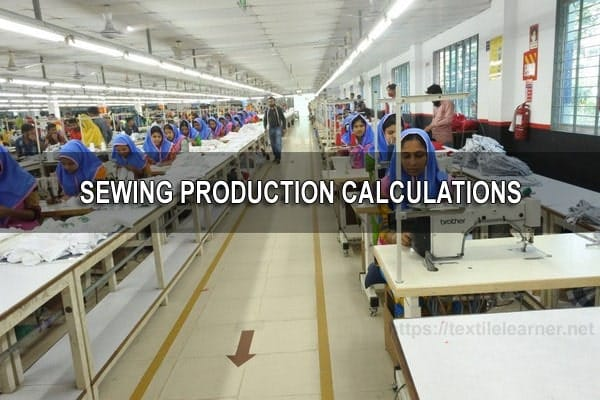# Sewing Production Calculations in Garment Industry

Last Updated on 11/01/2021

### Sewing Production Calculations in Garment Industry

R.S. Balakumar
Associate Professor
Dept of Fashion Design & Arts
Hindustan University, Chennai, India
Email: rsbalakumar1953@gmail.com

I have already published some articles on sewing operation, sewing faults and defects, sewing machine maintenance, questions and answers on sewing for students. In this article I will discuss on sewing production calculations in garment industry.(1) To calculate the number of machine necessary for daily production quantity in each process.

The number of sewing machines necessary for each process,

…..Time for each process X daily production quantity
=  ………………………………………………………….………………………………
…………………….Work time per day

Example: For dart sewing

Process time …………………………..0.76 min.
Work time per shift …………………480 min.
Production quantity per shift….400 pcs.

……………………………………………………………………………………0.76 X 400
So, The number of machines necessary for each process = …………………
……………………………………………………………………………………….480

The number machine necessary for sewing darts = 0.63 = 1 machine (ANS)

(2) The number of the machine you need will be calculated by applying the value obtained in (1) to the configuration (process composition).

In each park and each kind of machine. When you establish process composition, consideration to the layout which allows the works in process to run smoothly between lines must be taken.

(3) Calculation method of the number of the required machine according to the different of production quantity per shift or the number of persons

The number of required machine should be originally calculated in the way as above-stated in (1).

But there is a simplified calculation method by using MACHNE LIST and BASIC DATA described in this document.

(4) Calculation method of the number of required machines according to the difference of production quantity per shift

The number of required machine,

…………………………………………………..Production quantity per shift after change
= The number of each machine X ………………………………………………………………………
…………………………………………………………….Production quantity per shift

Example:

The number of DDL-555ON-7 –WB/CP230/AK-85…………………8 machines
Production quantity per shift …………………………………………200 pcs.
Production quantity per shift after change…………………………….120 pcs.

……………………………………………………………………….120 pcs
The number of required machine = 8 machine X …………
……………………………………………………………………….200 pcs

= 4.8 = 5 machine (ANS)

(5) Calculation method of the number of required machine according to the difference of persons
The number of required machine,

…………………………………………………The machine of persons per shift after change
= The number of each machine X ………………………………………………………………………..
……………………………………………………………………The number of person

Example:

The number of DDL-555ON-7-WB/CP230/AK-85 …………8 machines
Number of persons per shift ………….………………….45 persons
Number of persons per shift after change……………………100 persons

………………………………………………………………………….100 persons
The number of required machines = 8 machine X ……………………………………
…………………………………………………………………………..45 persons

= 17.7 = 18 machines (ANS)

(6) Calculation method of the production quantity per year according to the different of work days per year.  The production quantity per year is calculated on the basis of 280 days per year in this document. When the number of work days per year is different, use the following formula to obtain the production per year.

The production quantity per year,

……………………………………Work days per year after change
= Production per year X …………………………………………………………………
…………………………………………………..280 days

EXAMPLE:

Production quantity per year …………………..……………56,000 PCS.
Work days per year after change ……………………………250 days

…………………………………………………………………………..250 days
The production quantity per year = 56,000 pcs. X …………………..
………………………………………………………………………….280 days

= 50,000 pcs . (ANS)

(7) Method of the number of persons according to the difference of production quantity per shift

…………………………………………………………………Production quantity per shift after change
The number of persons = Number of persons X ………………….…………………..…………..……
…………………………………………………………………………Production quantity per shift

EXAMPLE:

The number of persons ……………………………..45 Persons
Production quantity per shift …………………….500 pcs.
Production quantity per shift after change ………………………850 pcs.

………………………………………………………….850 pcs.
The number of persons = 45 persons X ………………..……
…………………………………………………………500 pcs.

= 76.5 = 77 persons (ANS)

(8) Calculation method of production quantity per shift according to the different of the number of persons

From (formula 2),

Production quantity per shift,

…………………………………………………..The number of persons after change
= Production quantity per shift X ………………………………………………………..
………………………………………………………….The number of persons

EXAMPLE:

Production quantity per shift in BASIC DATA ………………500 pcs.
The number of persons in BASIC DATA ……………………….75 persons
The number of person after change ……………………………100 persons

…………………………………………………………………….100 persons
The production quantity per shift = 500 pcs. X ……………………
…………………………………………………………………….75 persons

= 666.7 =  666 pcs. (ANS)

(9) Simplified sewing production calculations method of the required floor space according to the difference of total number of required equipment

The required floor space = (required floor space per 1 finishing equipment X total number of sewing equipment) + (required floor space per 1 finishing equipment X total number of finishing equipment)

You may also like: How to Improve Productivity and Saving Cost in Sewing Department

EXAMPLE:

Required floor space per 1 sewing equipment …………..about 6 m2
Total number of sewing equipment ……………………………187 machines
Required floor space per 1 finishing equipment …………about 10 m2
Total number of finishing equipment ………………………….20 machines

Required floor space,

= (6 m2 /1 machine X 187 machine) + (10 m2 /1 machine X 20 machines)
= 1,322 m2 (ANS)

Reference:

BINRAN SYSTEM-JUKI LABORATORIES, JAPAN.

You may also like: